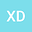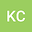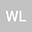Research on Definite Solution Problem of Partial Differential Equations under Two Kinds of Elastic Coefficients of External Boundary Conditions
•••• +3Kaitao Cui
The Second Research Institute of Civil Aviation Administration of China
Author ProfileWenjing Li
Xihua University
Author ProfileZheng Zeng
Xihua University
Author ProfileQun Liu
Xihua University
Author ProfileShun Li
Xihua University
Author Profile## Abstract

This paper studies the definite solution problems of partial differential equation (PDE) under two kinds of elastic coefficients (exponential function type, polynomial function type) of external boundary conditions. Then the definite solution problems are solved by Laplace transformation, the method of undetermined coefficients and Gaver-Stehfest numerical inversion equation. Firstly, the definite solution problems of linear PDE are transformed into the boundary value problems of linear differential equations in Laplace Space by Laplace transformation. Secondly, the solutions in Laplace space of the boundary value problems of linear differential equations are obtained through using the method of undetermined coefficients. Finally, Obtain the real space solution of the definite solution of PDE under three kinds of elastic coefficients of external boundary conditions by using the Gaver-Stehfest numerical inversion equation. According to the above solution steps, this paper gives two different examples and obtains the corresponding curve analysis of the definite solution problems of PDE under two kinds of elastic coefficients of external boundary conditions. The introduction of two kinds of elastic coefficients of external boundary conditions not only expands the scope of the research on the definite solution of PDE, but also improves the matching degree between the theoretical model and the actual problems.